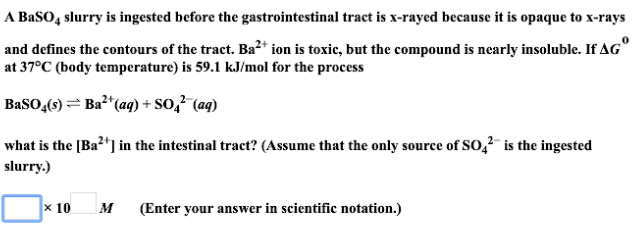# A BaSO4 slurry is ingested before the gastrointestinal tract is x-rayed because it is opaque to x-rays and defines the contours of the tract. Ba^2+ ion is toxic, but the compound is nearly insoluble. If ΔG° at 37°C (body temperature) is 59.1 kJ/mol for the process BaSO4(s) ⇌ Ba^2+(aq) + SO4^2-(aq) what is the [Ba^2+] in the intestinal tract? (Assume that the only source of SO4^2- is the ingested slurry.) (Enter your answer in scientific notation.)